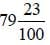# Test: Decimals- 1

## 10 Questions MCQ Test Mathematics for Class 4 | Test: Decimals- 1

Description
Attempt Test: Decimals- 1 | 10 questions in 15 minutes | Mock test for Class 4 preparation | Free important questions MCQ to study Mathematics for Class 4 for Class 4 Exam | Download free PDF with solutions
QUESTION: 1

### Write in number = Eighteen points five.

Solution:

18.5

QUESTION: 2

Solution:

0.27 + 0.67 = 0.94

QUESTION: 3

### Write in number name 86.07

Solution:

eighty six point zero seven.

QUESTION: 4

Write in rupees = 1063 rupees 75 P

Solution:

1063 rupees 75 P in Rupees is Rs. 1063.75

QUESTION: 5

Write the decimals number for 48/100

Solution:

Because 100 has two zeroes , so we'll write point in front of two digits.

0.48

QUESTION: 6

Rename the following as hundredth - 0.8

Solution:

0.8 as hundredth will be 0.80

QUESTION: 7

Write the following decimals as mixed numerals = 79.23

Solution:

79.23 =QUESTION: 8

Subtract 18.72 from 40

Solution:

40 - 18.72 = 21.28

QUESTION: 9

Write in rupees →3 rupees and 45 P

Solution:

3 rupees and 45 P = Rs. 3.45

QUESTION: 10

Subtract 7.03 – 2.76

Solution:

7.03 – 2.76 = 4.27Use Code STAYHOME200 and get INR 200 additional OFF Use Coupon Code